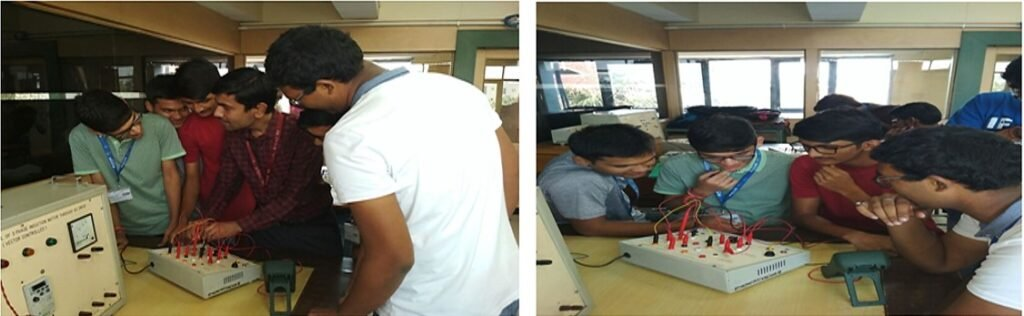Lab Room No:  A-304

Lab In charge:  Dr. SANDEEP SHARMA

Lab Assistant:  Mr. Ankit

Lab Subjects (Subject Code):   Circuit & System Lab (ETEE-207)

The Circuits & Systems laboratory has 5 fully equipped setup benches to carry out numerous fundamental experiments. The experiments are designed to expose students to the practical executions of the fundamental theories of Electrical Engineering.  The course is intended to enhance the students’ understanding of important analytical principles by engaging them in the real-world application of these principles in the laboratory.  An equally important purpose of the course is to further develop the students’ laboratory practice for experimentally testing and evaluating electrical circuits and systems. The lab is equipped with all modern experimental kits like Z, Y, ABCD parameter kit and series, parallel, cascade connection kit, multi-meter.  The zero printed circuit boards (PCBs) are also available with all electrical circuit components to fabricate any given circuit.Hardware Details: Experimental kits to calculate two port network parameter-Z, Y, H & Transmission parameter, interconnection of two 2-port network, Ammeter, Voltmeter, Power Supply, MATLAB installed 2 computers (Core I5,8GB RAM).

Software Detail: MATLAB

List of Experiment:

1. Introduction to MATLAB & its basic components.
2. Plot unit step, unit impulse, unit ramp, exponential, parabolic function and sinusoidal signals.
3. To determine Z and Y parameters of the given two port network.
4. To determine ABCD parameters of the given two port network.
5. To determine Hybrid parameters of the given two port network.
6. To design cascade connection and determine ABCD parameters of the given two port network.
7. To design series-series connection and determine Z parameter of the given two port network.
8. To determine parallel – parallel connection and determine Y parameter of the given two port
9. Study the transient response of series RLC circuit for different types of waveforms using
10. To verify various theorems in ac circuits.